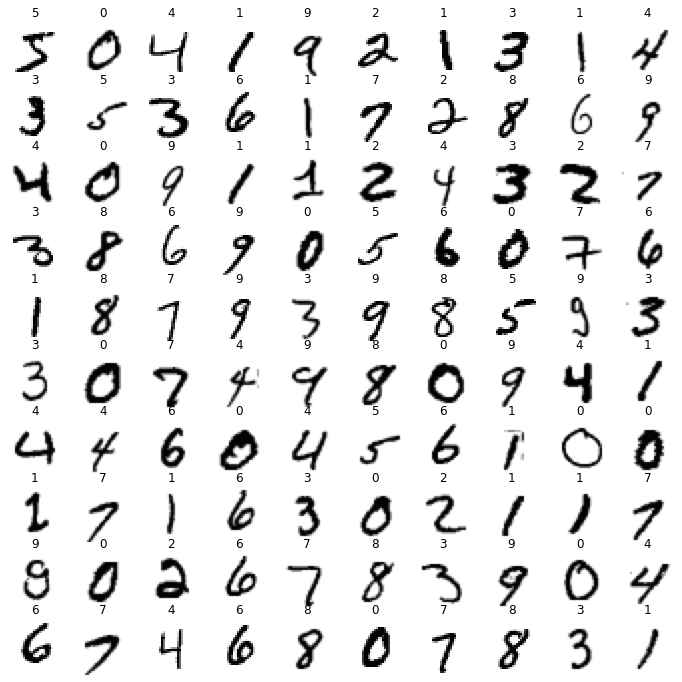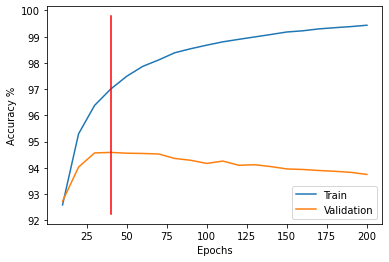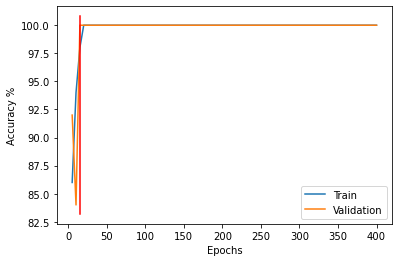# A4 Neural Network Classifier¶

## NeuralNetworkClassifier¶

Starting with the NeuralNetwork class defined in Lecture Notes 12, complete the subclass NeuralNetworkClassifier as discussed.

## percent_correct¶

When trying to classify real data, we need a way to evaluate our performance. One way is to just calculate the percent of samples correctly classified, and to show a confusion matrix. Define the function percent_correct(Y, T), that returns the percent of samples correctly classified, given T as a column matrix of class labels, and Y as the column matrix of classes predicted by use.

In :
import numpy as np

In :
X = np.array([[0, 0], [1, 0], [0, 1], [1, 1]])
T = np.array([, , , ])
X, T

Out:
(array([[0, 0],
[1, 0],
[0, 1],
[1, 1]]),
array([,
,
,
]))
In :
np.random.seed(111)
nnet = NeuralNetworkClassifier(2, , 2)

In :
nnet.Ws

Out:
[array([[ 0.12952296, -0.38212533, -0.07383268,  0.31091752, -0.23633798,
-0.40511172, -0.55139454, -0.09211682, -0.30174387, -0.18745848],
[ 0.56662595, -0.3028474 , -0.48359706,  0.19583749,  0.13999926,
-0.26066957, -0.03900416, -0.44067096, -0.49195143,  0.46277416],
[ 0.33943873,  0.39325596,  0.36397022,  0.56690583,  0.08922813,
0.36230683, -0.09085429, -0.5456561 , -0.05295844, -0.45573018]]),
array([[ 0.19129089,  0.11923431],
[ 0.03936858, -0.13614606],
[ 0.30059098, -0.21826885],
[ 0.06959828, -0.00902345],
[-0.05727085,  0.13739817],
[-0.10684722, -0.05997329],
[-0.10916737,  0.26968491],
[ 0.25249064,  0.18925528],
[-0.28096209,  0.2673639 ],
[ 0.27162504,  0.18488136],
[-0.01128977,  0.2814664 ]])]

If you add some print statements in neg_log_likelihood functions, you can compare your output to the following results.

In :
nnet.train(X, T, 1, 0.1, method='sgd')

In neg_log_likelihood: arguments are
X (standardized):
[[-1. -1.]
[ 1. -1.]
[-1.  1.]
[ 1.  1.]]
T (indicator vars):
[[1 0]
[0 1]
[0 1]
[1 0]]

In neg_log_likelihood: result of call to self.forward is:
[array([[-0.65071726, -0.44024437,  0.04576217, -0.42339865, -0.43460927,
-0.46740829, -0.39822372,  0.71346703,  0.23848393, -0.19208626],
[ 0.34231299, -0.79254131, -0.72655903, -0.06007838, -0.18346578,
-0.77314041, -0.46175878,  0.0128676 , -0.62959015,  0.62370478],
[-0.09735492,  0.30405172,  0.64909581,  0.5928089 , -0.27947188,
0.2144819 , -0.53935438, -0.19458859,  0.13639376, -0.80263068],
[ 0.77613968, -0.28371417, -0.19108161,  0.79083647, -0.00711046,
-0.294489  , -0.59233336, -0.79262132, -0.68931726, -0.1784822 ]]), array([[0.4190728 , 0.5809272 ],
[0.42642212, 0.57357788],
[0.59859207, 0.40140793],
[0.59715275, 0.40284725]])]

In neg_log_likelihood: result of np.log(Y + sys.float_info.epsilon) is:
[[-0.86971064 -0.54312982]
[-0.85232554 -0.55586155]
[-0.51317492 -0.9127771 ]
[-0.51558234 -0.90919781]]

neg_log_likelihood returns:
0.3567414532031946
sgd: Epoch 1 Error=0.69995

Out:
NeuralNetwork(2, , 2)
In :
np.exp(-0.35674)

Out:
0.6999544622383824

Now if you comment out those print statements, you can run for more epochs without tons of output.

In :
np.random.seed(111)
nnet = NeuralNetworkClassifier(2, , 2)

In :
nnet.train(X, T, 100, method='scg')

SCG: Epoch 10 Error=0.99066
SCG: Epoch 20 Error=0.99994
SCG: Epoch 30 Error=1.00000

Out:
NeuralNetwork(2, , 2)
In :
nnet.use(X)

Out:
(array([,
,
,
]),
array([[9.99999987e-01, 1.28250653e-08],
[1.01010817e-08, 9.99999990e-01],
[8.80184466e-09, 9.99999991e-01],
[9.99999979e-01, 2.13188420e-08]]))
In :
percent_correct(nnet.use(X), T)

Out:
100.0

Works! The XOR problem was used early in the history of neural networks as a problem that cannot be solved with a linear model. Let's try it. It turns out our neural network code can do this if we use an empty list for the hidden unit structure!

In :
nnet = NeuralNetworkClassifier(2, [], 2)
nnet.train(X, T, 100)   # default method is 'scg'

Out:
NeuralNetwork(2, [], 2)
In :
nnet.use(X)

Out:
(array([,
,
,
]),
array([[0.5, 0.5],
[0.5, 0.5],
[0.5, 0.5],
[0.5, 0.5]]))
In :
percent_correct(nnet.use(X), T)

Out:
50.0

A second way to evaluate a classifier is to calculate a confusion matrix. This shows the percent accuracy for each class, and also shows which classes are predicted in error.

Here is a function you can use to show a confusion matrix.

In :
import pandas

def confusion_matrix(Y_classes, T):
class_names = np.unique(T)
table = []
for true_class in class_names:
row = []
for Y_class in class_names:
row.append(100 * np.mean(Y_classes[T == true_class] == Y_class))
table.append(row)
conf_matrix = pandas.DataFrame(table, index=class_names, columns=class_names)
print('Percent Correct')

In :
confusion_matrix(nnet.use(X), T)

Percent Correct

Out:
0 1
0 50.0 50.0
1 50.0 50.0

## Apply NeuralNetworkClassifier to Images of Handwritten Digits¶

Apply your NeuralNetworkClassifier to the MNIST digits dataset.

In :
import pickle
import gzip

In :
with gzip.open('mnist.pkl.gz', 'rb') as f:
train_set, valid_set, test_set = pickle.load(f, encoding='latin1')

Xtrain = train_set
Ttrain = train_set.reshape(-1, 1)

Xval = valid_set
Tval = valid_set.reshape(-1, 1)

Xtest = test_set
Ttest = test_set.reshape(-1, 1)

print(Xtrain.shape, Ttrain.shape,  Xval.shape, Tval.shape,  Xtest.shape, Ttest.shape)

(50000, 784) (50000, 1) (10000, 784) (10000, 1) (10000, 784) (10000, 1)

In :
28*28

Out:
784
In :
def draw_image(image, label):
plt.imshow(-image.reshape(28, 28), cmap='gray')
# plt.axis('off')
plt.xticks([])
plt.yticks([])
plt.axis('off')
plt.title(label)

In :
plt.figure(figsize=(12, 12))
for i in range(100):
plt.subplot(10, 10, i+1)
draw_image(Xtrain[i], Ttrain[i,0])In :
nnet = NeuralNetworkClassifier(784, [], 10)
nnet.train(Xtrain, Ttrain, 40)

SCG: Epoch 4 Error=0.95202
SCG: Epoch 8 Error=0.96838
SCG: Epoch 12 Error=0.97241
SCG: Epoch 16 Error=0.97312
SCG: Epoch 20 Error=0.97312
SCG: Epoch 24 Error=0.97312
SCG: Epoch 28 Error=0.97326
SCG: Epoch 32 Error=0.97444
SCG: Epoch 36 Error=0.97531
SCG: Epoch 40 Error=0.97603

Out:
NeuralNetwork(784, [], 10)
In :
[percent_correct(nnet.use(X), T) for X, T in zip([Xtrain, Xval, Xtest], [Ttrain, Tval, Ttest])]

Out:
[93.362, 92.64, 92.13]
In :
nnet = NeuralNetworkClassifier(784, , 10)
nnet.train(Xtrain, Ttrain, 40)

SCG: Epoch 4 Error=0.93705
SCG: Epoch 8 Error=0.96538
SCG: Epoch 12 Error=0.97457
SCG: Epoch 16 Error=0.97976
SCG: Epoch 20 Error=0.98303
SCG: Epoch 24 Error=0.98530
SCG: Epoch 28 Error=0.98699
SCG: Epoch 32 Error=0.98836
SCG: Epoch 36 Error=0.98957
SCG: Epoch 40 Error=0.99070

Out:
NeuralNetwork(784, , 10)
In :
[percent_correct(nnet.use(X), T) for X, T in zip([Xtrain, Xval, Xtest],
[Ttrain, Tval, Ttest])]

Out:
[97.492, 94.08, 93.33]

## Define train_for_best_validation and apply to MNIST data¶

Using the function run from Lecture Notes 11 as a guide, define a new function train_for_best_validation that accepts arguments

• Xtrain, Ttrain: matrices of shapes $N\times D$ and $N\times 1$ as input and target training data, where $N$ is number of training samples and $D$ is number of input components,
• Xval, Tval: matrices of shapes $N\times D$ and $N\times 1$ of validation data ($N$ not same value as above)
• n_epochs: total number of epochs to train for,
• n_epochs_per_train: divide n_epochs by this value to get number of times the neural network train function is called for this many epochs,
• n_hiddens_list: structure of hidden layers,
• method: optimizer method,
• learning_rate: used for optimizer methods 'adam' and 'sgd'.

It must return

• nnet: resulting neural network with weights that produced the highest accuracy for the validation data set,
• epoch: epoch corresponding to best validation accuracy,
• train_accuracy: accuracy at that best epoch on training data,
• val_accuracy: accuracy at that best epoch on validation data.

This function should call your percent_correct function to calculate classification accuracies.

Apply it to the MNIST data as shown below.

In :
nnet, epoch, train_accuracy, val_accuracy = train_for_best_validation(Xtrain, Ttrain,  Xval, Tval,
200, 10, , method='scg')

Epoch 10: Train Accuracy 92.58800000000001 Validation Accuracy 92.73
Epoch 20: Train Accuracy 95.298 Validation Accuracy 94.03
Epoch 30: Train Accuracy 96.382 Validation Accuracy 94.57
Epoch 40: Train Accuracy 97.0 Validation Accuracy 94.59
Epoch 50: Train Accuracy 97.49 Validation Accuracy 94.56
Epoch 60: Train Accuracy 97.868 Validation Accuracy 94.55
Epoch 70: Train Accuracy 98.114 Validation Accuracy 94.53
Epoch 80: Train Accuracy 98.38799999999999 Validation Accuracy 94.36
Epoch 90: Train Accuracy 98.542 Validation Accuracy 94.28999999999999
Epoch 100: Train Accuracy 98.678 Validation Accuracy 94.17
Epoch 110: Train Accuracy 98.804 Validation Accuracy 94.26
Epoch 120: Train Accuracy 98.9 Validation Accuracy 94.1
Epoch 130: Train Accuracy 98.992 Validation Accuracy 94.12
Epoch 140: Train Accuracy 99.084 Validation Accuracy 94.05
Epoch 150: Train Accuracy 99.18 Validation Accuracy 93.96
Epoch 160: Train Accuracy 99.226 Validation Accuracy 93.94
Epoch 170: Train Accuracy 99.298 Validation Accuracy 93.89999999999999
Epoch 180: Train Accuracy 99.344 Validation Accuracy 93.87
Epoch 190: Train Accuracy 99.386 Validation Accuracy 93.83
Epoch 200: Train Accuracy 99.438 Validation Accuracy 93.75
Best validation accuracy is at epoch 40.0 with Accuracy Train of 97.00% and Validation of 94.59%In :
[percent_correct(nnet.use(X), T) for X, T in zip([Xtrain, Xval, Xtest], [Ttrain, Tval, Ttest])]

Out:
[97.0, 94.59, 93.74]

Call train_for_best_validation a number of times to compare accuracies using the three different optimization methods, and a few different values of n_epochs, n_epochs_per_train, and n_hiddens_list, and learning_rate when using 'sgd' and 'adam'. You do not have to find the very best values of these parameters. For example, for n_hiddens_list at least use [] (a linear model) and a larger network, like [100, 100].

Show the confusion matrix for the network that gave you the best validation accuracy.

Write at least 10 sentences about what you observe in the accuracy plots, the train, validation and test accuracies, and the confusion matrix.

Download A4grader.tar, extract A4grader.py before running the following cell.

In :
%run -i A4grader.py

======================= Code Execution =======================

Extracting python code from notebook named 'Anderson-A4.ipynb' and storing in notebookcode.py
Removing all statements that are not function or class defs or import statements.

## Testing constructor ####################################################################

# Linear network
nnet = NeuralNetworkClassifier(2, [], 5)
# Is isinstance(nnet, NeuralNetwork) True?

--- 5/5 points. NeuralNetworkClassifier is correctly of type NeuralNetwork

## Testing constructor ####################################################################

# Linear network
nnet = NeuralNetworkClassifier(2, [], 5)
W_shapes = [W.shape for W in nnet.Ws]

--- 5/5 points. W_shapes is correct value of [(3, 5)]

## Testing constructor ####################################################################

G_shapes = [G.shape for G in nnet.Gs]

--- 5/5 points. G_shapes is correct value of [(3, 5)]

## Testing train ####################################################################

np.random.seed(42)
X = np.random.uniform(0, 1, size=(100, 2))
T = (np.abs(X[:, 0:1] - X[:, 1:2]) < 0.5).astype(int)

nnet = NeuralNetworkClassifier(2, [10, 5], 2)
nnet.train(X, T, 1000, method='scg')

Then check  nnet.get_error_trace()

SCG: Epoch 100 Error=0.99999

--- 10/10 points. Correct values in error_trace

## Testing train ####################################################################

np.random.seed(42)
X = np.random.uniform(0, 1, size=(100, 2))
T = (np.abs(X[:, 0:1] - X[:, 1:2]) < 0.5).astype(int)

nnet = NeuralNetworkClassifier(2, [10, 5], 2)
nnet.train(X, T, 1000, method='scg')
classes, prob = nnet.use(X)

SCG: Epoch 100 Error=0.99999

--- 10/10 points. Correct values in classes

--- 10/10 points. Correct values in prob

## Testing percent_correct ####################################################################

Y = np.array([1, 2, 3, 1, 2, 3]).reshape(-1, 1)
T = np.array([1, 2, 3, 3, 2, 1]).reshape(-1, 1)
pc = percent_correct(Y, T)

--- 5/5 points. Correct percent_correct.

## Testing train_for_best_validation ####################################################################

np.random.seed(42)
X = np.random.uniform(0, 1, size=(100, 2))
np.random.shuffle(X)
T = (np.abs(X[:, 0:1] - X[:, 1:2]) < 0.5).astype(int)

Xtrain = X[:50, :]
Ttrain = T[:50, :]
Xval = X[50:75, :]
Ttest = T[50:75, :]
Xtest = X[75:, :]
Ttest = T[75:, :]

nnet, epoch, train_accuracy, val_accuracy = train_for_best_validation(Xtrain, Ttrain,  Xval, Tval,
400, 10, [10, 10], method='scg')

Best validation accuracy is at epoch 15.0 with Accuracy Train of 98.00% and Validation of 100.00%

--- 5/5 points. Correct best epoch.

--- 5/5 points. Correct train_accuracy.

--- 5/5 points. Correct val_accuracy.

======================================================================
A4 Execution Grade is 65 / 65
======================================================================

__ / 10 points. Correctly applied train_for_best_validation function
to MNIST data.

__ / 5 points. Experimented with different values of parameters as
arguments to train_for_best_validation.

__ / 5 points. Show confusion matrix for best neural network.

__ / 10 points. Described results with at least 10 sentences.

======================================================================
A4 Results and Discussion Grade is ___ / 35
======================================================================

======================================================================
A4 FINAL GRADE is  _  / 100
======================================================================

Extra Credit: Earn one point of extra credit for repeating the above
experiments for another classification data set.

A4 EXTRA CREDIT is 0 / 1# Extra Credit¶

Repeat the above experiments with a different data set. Randonly partition your data into training, validaton and test parts if not already provided. Write in markdown cells descriptions of the data and your results.